# 羨慕實時資料看板？來看看Python的互動資料分析視覺化工具！ ⛵

## highlight: a11y-dark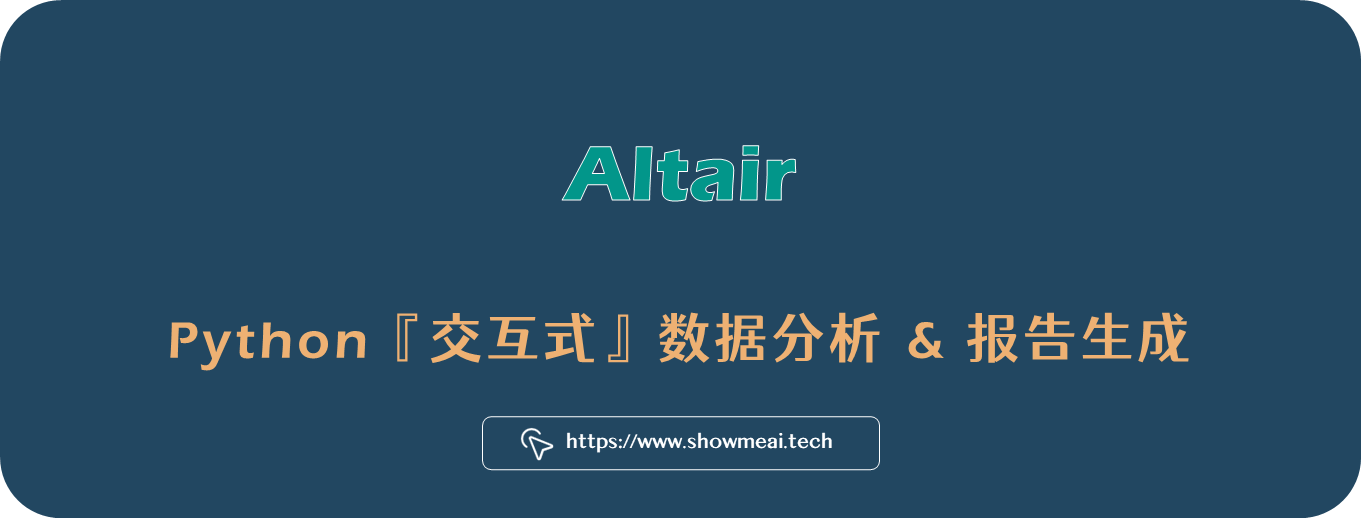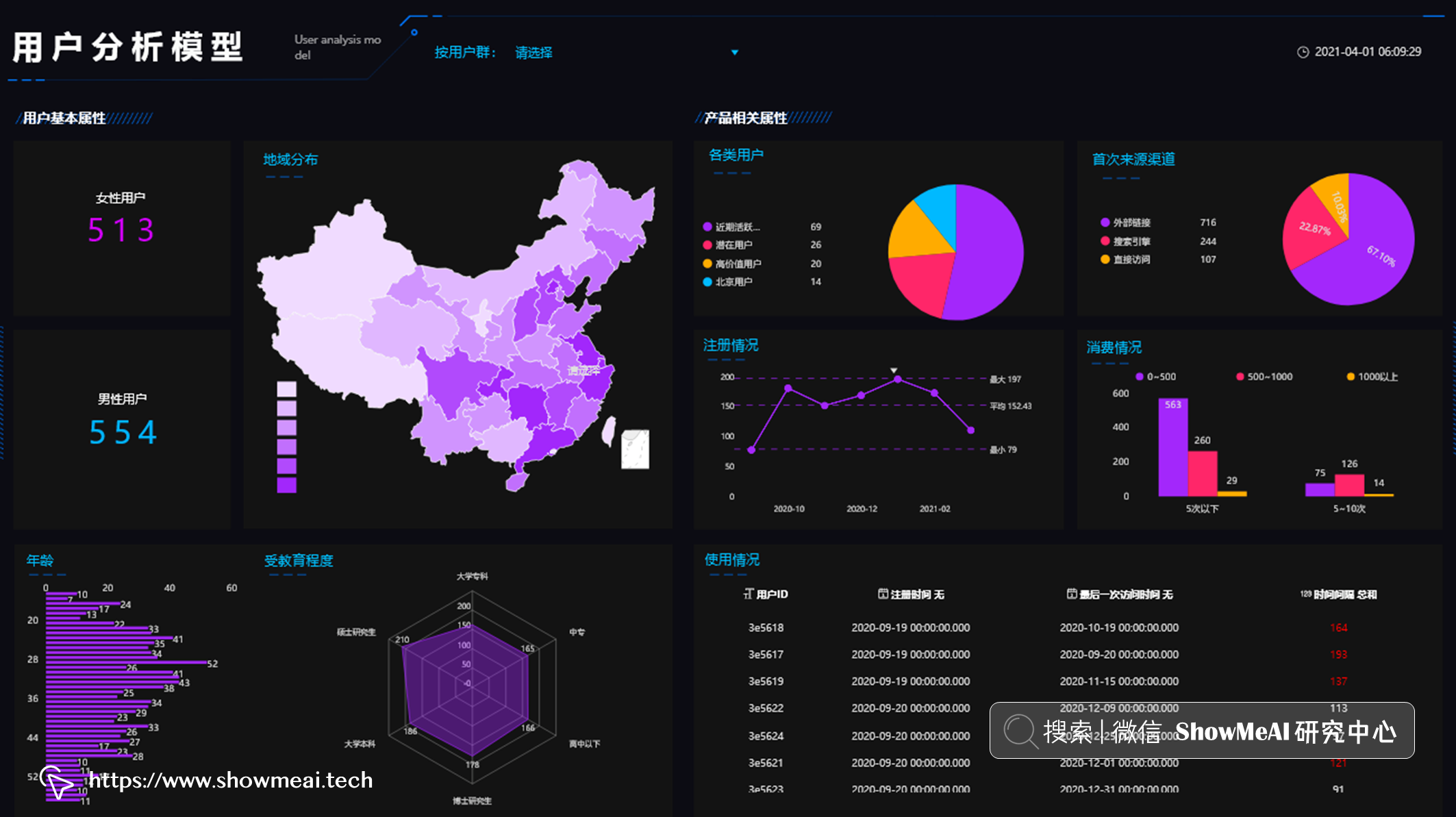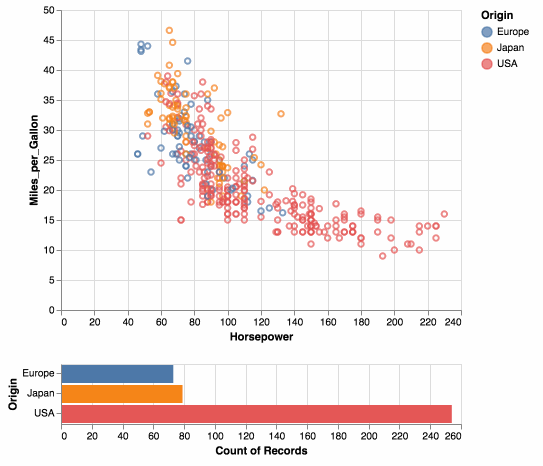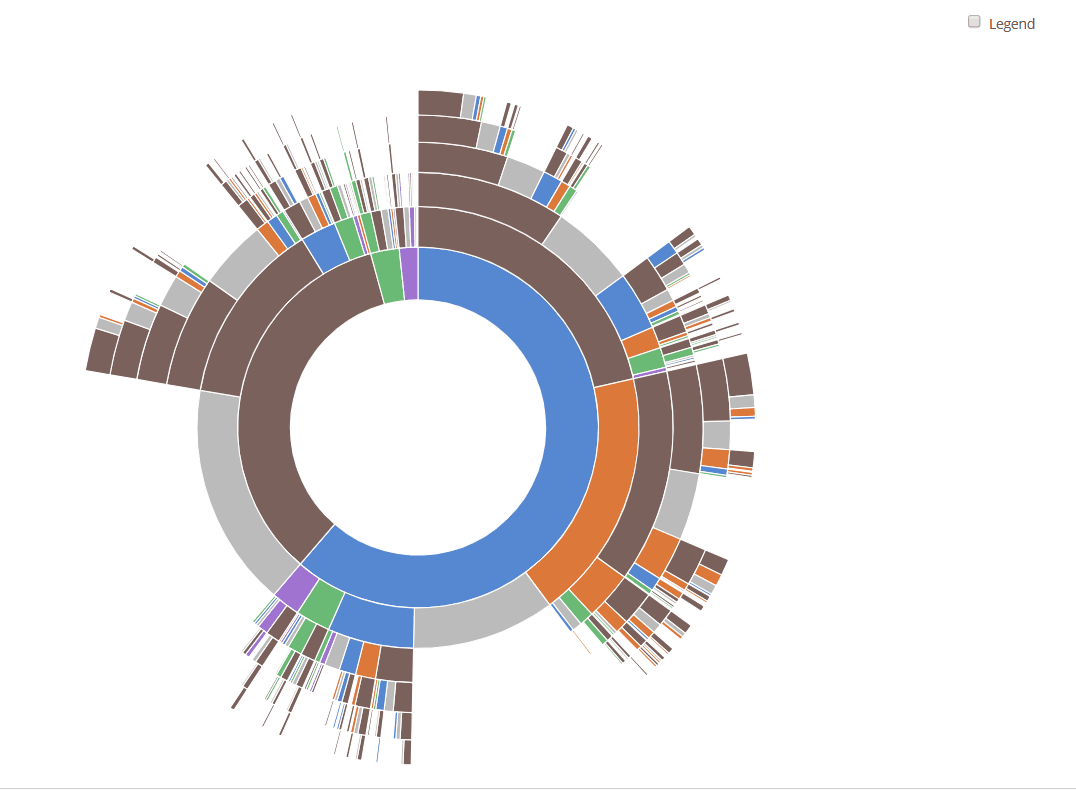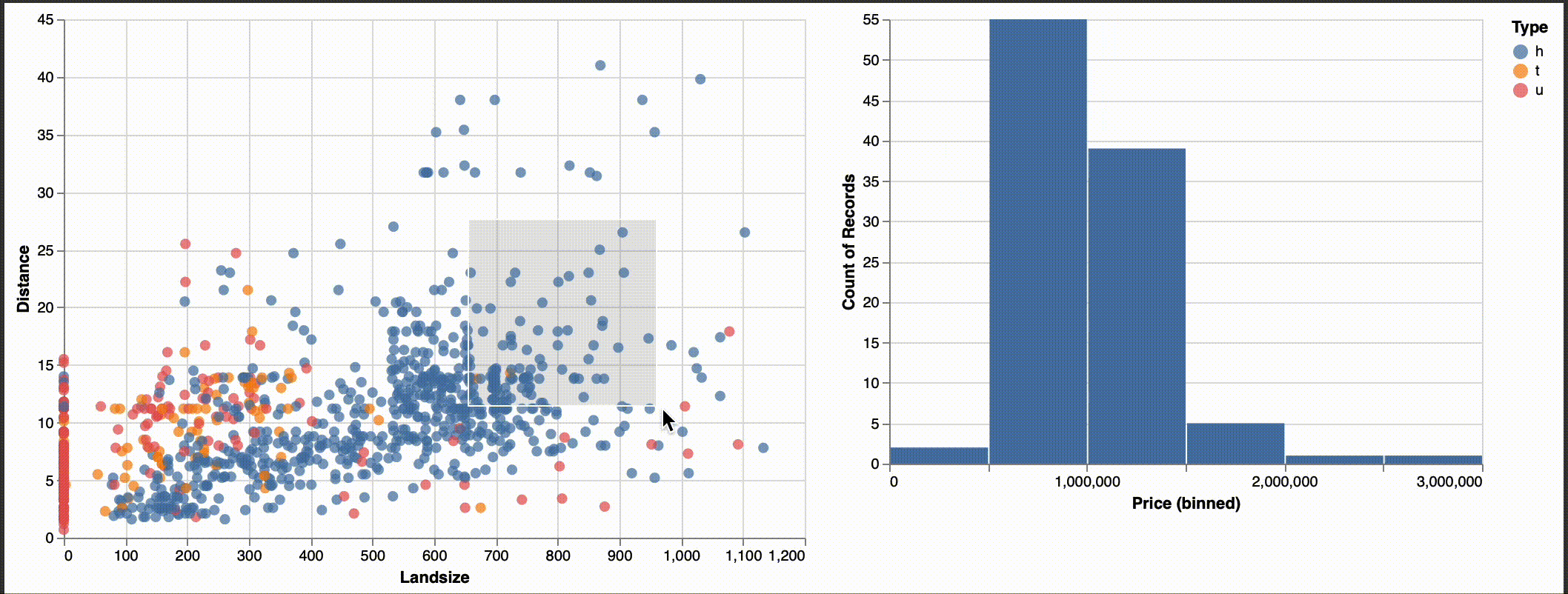```python

# 匯入工具庫

import altair as alt import pandas as pd

# 忽律資料規模限制

alt.data_transformers.enable('default', max_rows=None)

# 構建chart函式，它讀取資料和欄位名稱，返回一個互動式圖表結果

def chart(dataset, column_name, target_var): w = 500 single = alt.selection_single()

``````# 灰度圖與柱狀圖

# 如果是類別型欄位，我們不用分桶
if (column_name in dataset.select_dtypes(include='object').columns.to_list()):
a = alt.Chart(dataset).mark_bar().encode(
alt.X(column_name + ':N', bin=False),
alt.Y('count()'),
color = alt.condition(single, alt.value('#4c78a8'), alt.value('lightgray')),
tooltip=['count()', alt.Tooltip(column_name, bin=False)]

# 如果是數值型欄位，我們先分桶
else:
a = alt.Chart(dataset).mark_bar().encode(
alt.X(column_name + ':Q', bin=True),
alt.Y('count()'),
color = alt.condition(single, alt.value('#4c78a8'), alt.value('lightgray')),
tooltip=['count()', alt.Tooltip(column_name, bin=True)]

# 對於類別型欄位，我們構建它和目標欄位的一個箱線圖表；對於數值型欄位，我們構建它們和目標欄位的散點分佈圖
try:
if (column_name in dataset.select_dtypes(include='object').columns.to_list()):
b = alt.Chart(dataset).mark_boxplot().encode( #, title="Boxplot of " + column_name
alt.X(column_name + ':N'),
alt.Y(target_var),
color = alt.condition(single, alt.value('#4c78a8'), alt.value('lightgray')),
tooltip=[target_var]
else:
b = alt.Chart(dataset).mark_point().encode(
alt.X(column_name + ':Q'),
alt.Y(target_var),
color = alt.condition(single, alt.value('#4c78a8'), alt.value('lightgray')),
tooltip=[target_var]
except:
pass

return(a | b)
``````

```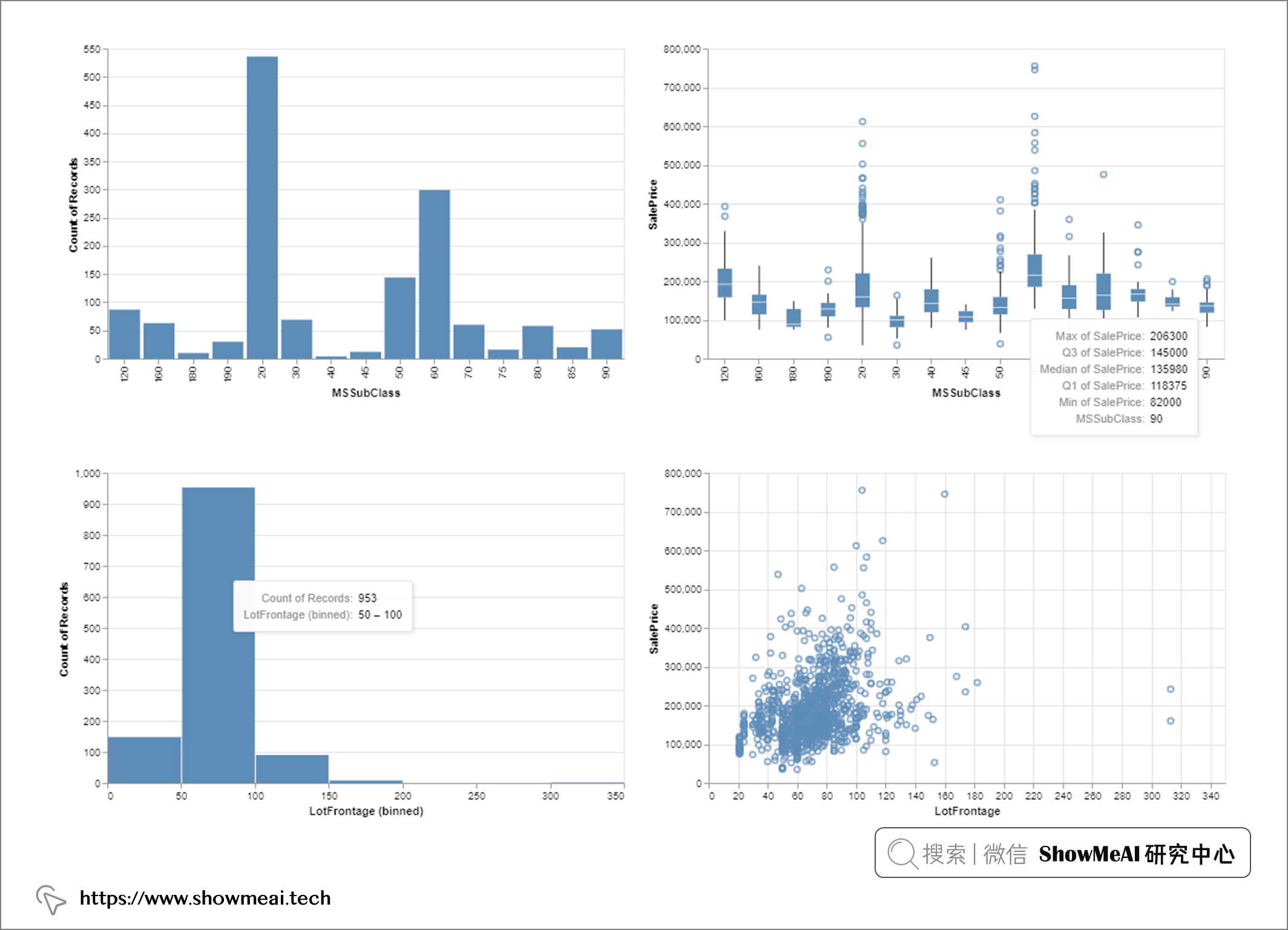```python

# 把所有的altair圖表新增到一個列表裡

myl = [] for col in dataset.columns: try: myl.append(chart(dataset, col, target_var)) except: pass else: pass

# 編譯所有的圖表到1個html檔案中

a = myl for i in range(1,len(myl)): a = a & myl[i] a.properties( title = 'Feature Histograms & Boxplots' ).configure_axis(labelFontSize=15, titleFontSize=25) a.save('figures/Feature_Visuals.html')

# 檢查是否所有的欄位都可以被視覺化

print('Features accounted for:', len(myl), 'out of', len(dataset.T)) ```

# 參考資料